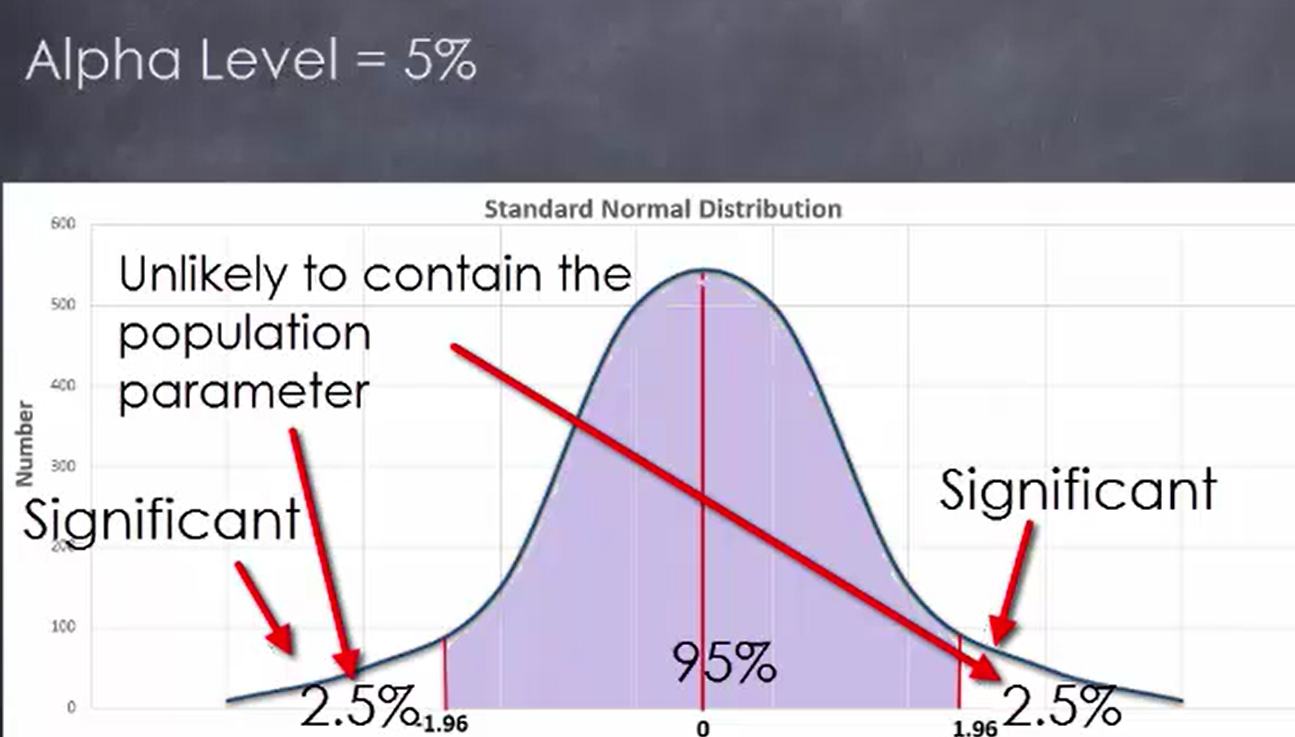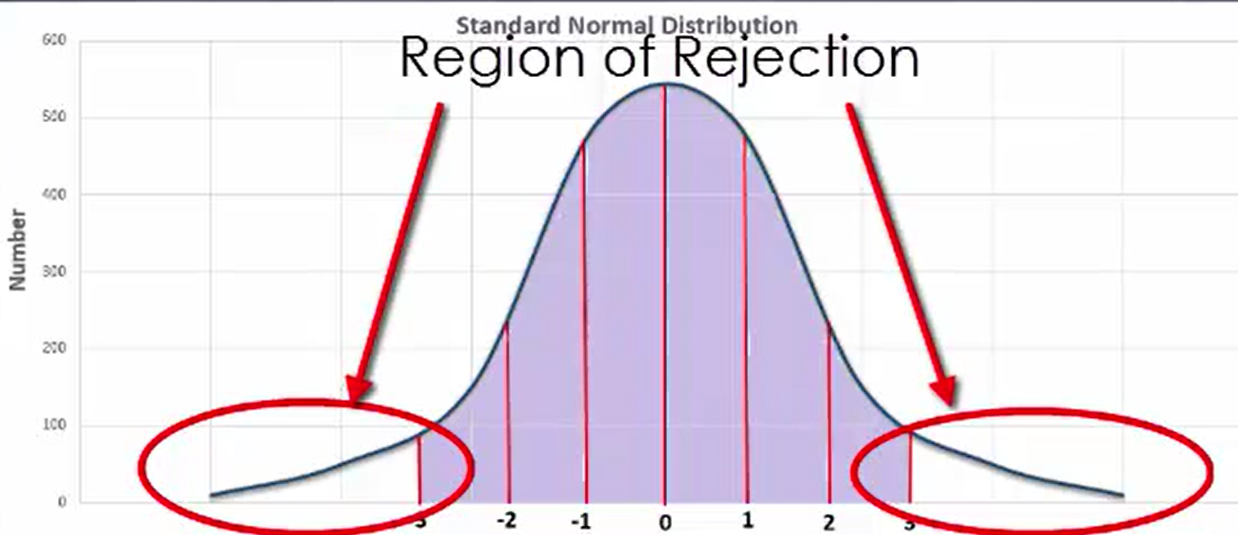+
Determining Significance
Interpret Inferential Statistics
0
of 0 possible points

# Determining Significance

Author: Sophia Tutorial
##### Description:

Identify key principles of statistical significance.

(more)

Sophia’s self-paced online courses are a great way to save time and money as you earn credits eligible for transfer to many different colleges and universities.*

No credit card required

37 Sophia partners guarantee credit transfer.

299 Institutions have accepted or given pre-approval for credit transfer.

* The American Council on Education's College Credit Recommendation Service (ACE Credit®) has evaluated and recommended college credit for 32 of Sophia’s online courses. Many different colleges and universities consider ACE CREDIT recommendations in determining the applicability to their course and degree programs.

Tutorial
what's covered
This lesson discusses determining significance. The first objective is to be able to understand when a null hypothesis can be rejected based on a region of rejection and a level of significance. The second objective is to understand the meaning of the alpha level and critical region. This lesson covers:
1. Hypotheses and Significance
2. Significance Level and Region of Rejection

## 1. Hypotheses and Significance

Recall from an earlier lesson that with the experimental method, the goal is to establish whether or not a cause-and-effect relationship exists between two variables. In this case, the null hypothesis states that a cause-and-effect relationship does not exist between two variables, while the alternative hypothesis states that such a cause-and-effect relationship could exist. The result of an experiment is considered significant when it is unlikely to occur, given the null hypothesis is true.

• Null Hypothesis: States that a cause and effect relationship does NOT exist between two variables.
• Alternative Hypothesis: States that a cause and effect relationship COULD exist between two variables.

When evaluating the results of an experiment, it is important to know if the results fall in the region of rejection so that you are able to reject the null hypothesis and suggests that the alternative hypothesis may be credible. Additionally, there are a variety of other uses for both the null and alternative hypotheses other than establishing whether or not a cause-and-effect relationship between two variables exists. However, you will not be covering these in this lesson.

## 2. Significance Level and Region of Rejection

A significance level indicates that part of a normal distribution contains values that are unlikely to contain the population parameter that you are estimating by engaging in random sampling. This level, also known as an alpha level, will always be equal to 5% and corresponds to results of experiment that are unlikely to occur when the null hypothesis is actually true. Results that fall in the region of rejection are considered to be significant. It is essential to identify when results fall in the region of rejection, as this tells us that the null hypothesis may be faulty.This region of rejection is based on the alpha level of 5%, which means that the rejection regions fall to the right of z = 1.96 and to the left of z = -1.96. In the event that one engages in many tests and realizes a substantial number of the results fall within the region of rejection, there may be reason to reject the null hypothesis. If an experiment’s results consistently fall within this region of rejection, the alternative hypothesis may have some validity as a result.

Consider the number of hours that a student spends preparing for a major exam and the score they receive on that exam. In this instance, the null hypothesis states that regardless of the amount of time one studies, it will not have an impact on the exam score. Since common sense tells us that studying does have an effect on how well one does on an exam, you would expect the results of such an experiment to fall within the region of rejection.As indicated in the graph shown here, the range of rejection falls in the tail area, so the results of the experiment regarding time spent studying and exam scores would be expected to fall in this area. In the event that one continues getting such results as the experiment is continued, there is reason to reject the null hypothesis and suggest that the alternative hypothesis might be valid. In the case of the studying experiment, such an alternative hypothesis could be that the amount of time one studies for an exam has an effect on exam success.

summary
This lesson looked at the relationship between hypotheses and significance. A null hypothesis can be rejected based upon results falling into the significance level and region of rejection.

Source: This work is adapted from Sophia author Dan Laub.

Rating